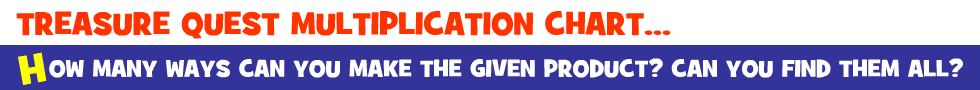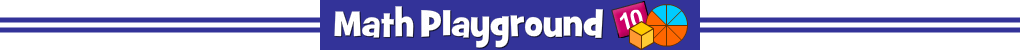Find the target product on the multiplication chart and tap the square.
You must find all possible answers.
The small number to the left of each orb shows how many squares to select.
There are 10 orbs to collect.
Treasure Quest Multiplication Chart - Learning Connections
Essential Skills
Mental Math - visualize multiplication patterns

Common Core Connections - Grades 3 and 4
Understand properties of multiplication.
Multiply within 100.
Identify arithmetic patterns.
Look for and make use of structure.
More Math Games to Play